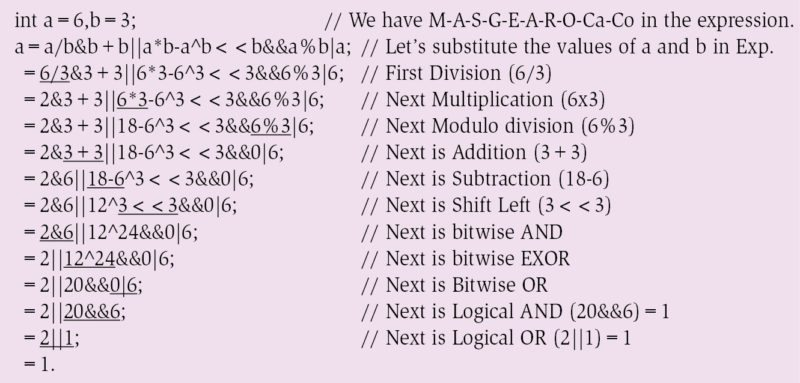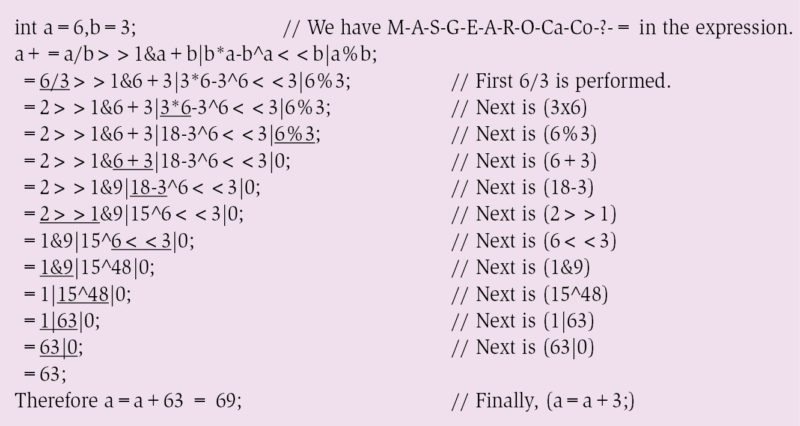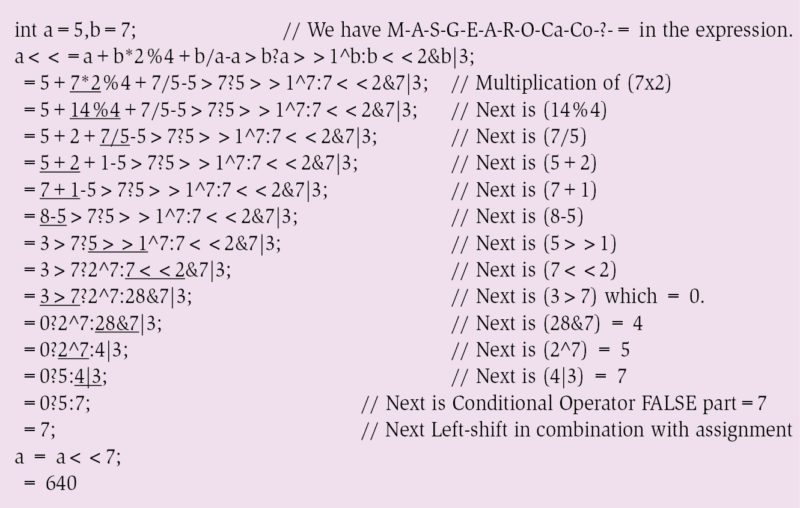Saturday, October 1, 2022

# The Rhyme Of C Operators

N. Abid Ali Khan is working as assistant professor in Department of ECE at Vasavi College of Engineering (autonomous) at Hyderabad. He has been working in the field of power-efficient embedded networking architectures, and is passionate about microcontrollers, ARM, RTOSes, embedded systems and programming

It is thanks to Dennis Ritchie and his team that the first C compiler was released way back in 1972. C language has found its own place in developing embedded system software, real-time operating system policy implementations and device drivers ranging from simple 8-bit microcontrollers to complex 32-bit FPGA targets. This article takes you through the basics of C-supported operators and describes an easy way to remember the priority of operators while evaluating an expression.

If you can remember the slogan ‘M-A-S-G-E-A-R-O-Ca-Co-?-=’ (left to right), any C expression can be calculated without actually typing the expression in a C compiler. Many books provide a standard table discussing the priority of operators and their associativity. But, when an expression is given, it is a little cumbersome to work it out by recalling the table all the time. This article also serves as an easy way to keep the order of priority on the fingertips.

Let us take the example of priority of execution of C expression shown in Fig. 2. This expression evaluation is simple; since only arithmetic operators are involved, where multiplicative operators (multiplication, division and modulo division) have high priority, while additive operators (addition and subtraction) have lower priority. If the expression is found to have operators of the same level of priority, evaluation starts from left to right (associativity). Step-by-step evaluation is also mentioned by specifying the high-priority operator with an underline.

Now, let us see how priority of the expression changes if we introduce bit-wise operators (bit-wise AND – &, bit-wise EXOR – ^ and bit-wise OR – |) in combination with arithmetic operators. For our use case, let us consider the expression shown in Fig. 3. Notice that, initially, multiplicative operators from left to right are evaluated. Then come additive operators; finally, when we land with only bit-wise operators, order of priority is first bit-wise AND, followed by bit-wise EXOR with bit-wise OR at the end. The expression, which has to be evaluated, is underlined for each step.

In the above expression, we missed the shift-left (<<) and shift-right (>>) operators. In fact, these are at the third position in the rhyme. Consider another expression given in Fig. 4, where multiplicative, additive and all bit-wise operators exist, including shift operators. This expression looks crispy, and no coder opts for it.

However, if you are asked to work on driver development with RAM memory organisation whose CPU registers are not bit-addressable, you may land up with expression(s) like this. As advised, you have to follow the order of the slogan, considering the priority with multiplicative→additive→shift-left or shift-right→bit-wise AND→bit-wise EXOR→bit-wise OR to evaluate the expression for arriving at the correct answer.

The shift-left and shift-right operators have significant improvements in terms of power because if you are shifting integer N by k times towards the left, you are multiplying N with 2k. On the other hand, if you are shifting N by k bits towards the right, you are dividing the number by 2k.

That means, for an integer N,

N<<k≡N×2k // Power-efficient multiplication

N>>k≡N/2k // Power-efficient division

Let us add relational operators of the two operands and try to get the value of the expression shown in Fig. 5 by following the priority order of the operator rhyme. Note that, within the available relational operators, there exist two priority levels among equality operators (low) and greater or less than operators (high).

Next, let us observe the behaviour of compound conditional AND (&&) and compound conditional OR (||) statements, too. Among these, logical AND (&&) has higher priority compared to logical OR (||). In the expression given in Fig. 6, again, by following the operator’s priority rhyme, the step-by-step value is obtained.

Assignment operator (=) is the last priority in the rhyme. Note that, other than the regular assignment operator, a combination of assignment with arithmetic operators or with bit-wise operators too may fall under this observation. For example, consider the expression in Fig. 7. After evaluating the complete expression on the left-hand side, 63 is the result.

Next, we have an assignment operator concatenated with an addition operator; this addition will be performed in the end, yielding the final value as 69.

Finally, consider the expression shown in Fig. 8, where most operators are deliberately used to understand how to evaluate to a final value, by following the order of priority as per the rhyme.Fig. 6: With multiplicative, additive, bit-wise and compound conditional (&& and ||) operatorsFig. 7: C expression with arithmetic, bit-wise (Shift, AND, EXOR, OR) and assignment operatorsFig. 8: C expression with arithmetic, bit-wise, relational, conditional and assignment operators

Although the expressions given in Figs 5, 6 and 7 are vague in nature—which a developer rarely adopts, for the other expressions, especially expressions that can be a combination of multiplicative, additive, bit-wise and assignment operators in a single expression, precedence of operators will come in force. As per the operator’s rhyme from left to right, value can be calculated.

#### 1 COMMENT

1.P bharath kumar

Sir,we are very much benefited from this article. please post more articles. So that we (students) can remember the orders and sequences.